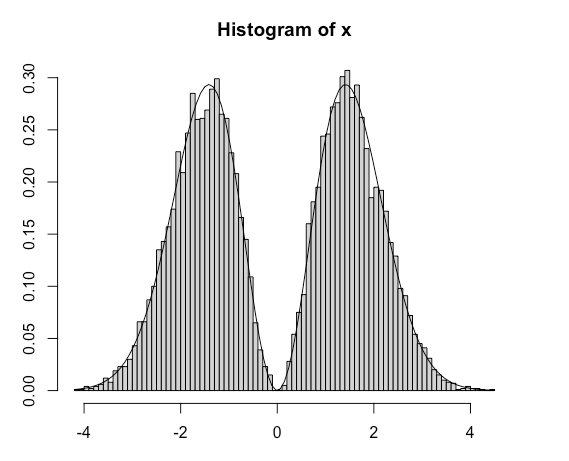A question that came out on X validated a few days ago is how to efficiently simulate from a distribution with density x²φ(x). (Obviously properly normalised since the second moment of the standard Normal  distribution is one.) The first solution that came out (by Jarle Tufto) exploits the fact that this density corresponds to a signed root of a χ²(3) variate. This is a very efficient proposal that requires a Gamma sampler and a random sign sampler. Since the cdf is available in closed form, Φ(x)-xφ(x), I ran a comparison with a numerical inversion, but this is much slower. I also tried an accept-reject version based on a Normal proposal with a larger variance, but even when optimising this variance, the running time was about twice as large. While checking Devroye (1986) for any possible if unlikely trick, I came upon this distribution twice (p.119 in an unsolved exercise, p.176 as the Maxwell distribution). With the remark that, if X~x²φ(x), Y=UX~φ(x). Inverting this result leads to X being distributed as sign(Y)√(Y^2-2log(U)), which recovers the original χ²(3) solution, if slightly increasing the simulation speed.